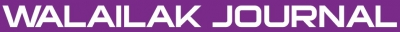### First Integral Method for Systems of (1+1)-Dimensional Dispersive Long Wave

#### Abstract

The First Integral method (FIM) is applied to solve a dispersive long wave system. In this method the division theorem, as a statement in commutative Algebra has an important role. To show the ability and the efficiency of this approach an example is provided. Application of FIM to the illustrative example leads to six exact solutions, which it is shown that these six solutions are independent to each other. So there are six different exact solutions.

doi:10.14456/WJST.2015.45

#### Keywords

First Integral method, exact solution, dispersive long wave (1+1)-dimensional systems, partial differential equation, non-linear algebraic equations

PDF

#### References

MR Miura. Backlund Transformation. Springer-Verlag, Berlin, 1978.

VB Matveev and MA Salle. Darboux Transformations and Solitons. Berlin, Heidelberg, Springer, 1991.

EJ Parkes and BR Duffy. An automated tanh-function method for finding solitary wave solution to nonlinear evolution equations. Comput. Phys. Comm. 1998; 98, 288-300.

AM Wazwaz. The extended tanh method for new soliton solutions for many forms of the fifth-order KdV equations. Appl. Math. Comput. 2007; 184, 1002-14.

SA Elwakil, SK EL-Labany, SK Zahran and MA Sabry. Modified extended tanh-function method for solving nonlinear partial differential equations. Phys. Lett. A 2002; 299, 179-88.

YT Gao and B Tian. Generalized hyperbolic-function method with computerized symbolic computation to construct the solitonic solutions to nonlinear equations of mathematical physics. Comput. Phys. Comm. 2001; 133, 158-64.

XY Tang and SY Lou. Localized excitations in (2+1)-dimensional systems. Phys. Rev. E 2002; 66, 46601-17.

ZS Feng. The first integral method to study the Burgers-Korteweg-de Vries equation. Phys. Lett. A 2002; 35, 343-9.

K Hosseini, R Ansari and P Gholamin. Exact solutions of some nonlinear systems of partial differential equations by using the first integral method. J. Math. Anal. Appl. 2012; 387, 807-14.

ZS Feng and XH Wang. The first integral method to the two-dimensional Burgers-Korteweg-de Vries equation. Phys. Lett. A 2003; 308, 173-8.

B Lu. The first integral method for some time fractional differential equations. J. Math. Anal. Appl. 2012; 395, 684-93.

MJ Ablowitz and PA Clarkson. Solitons Nonlinear Evolution Equations and Inverse Scattering. London Cambridge University Press, 1991.

TR Ding and CZ Li. Ordinary Differential Equations. Peking University Press, Peking, 1996.

N Bourbaki. Commutative Algebra. Addison-Wesley, Paris, 1971.

ME Minasari, D Ganji and MO Minasari. Jacobi elliptic function solutions of the (1+1) dimensional dispersive long wave equation by Homotopy Perturbation Method. Numer. Meth. Part. Differ. Equat. 2008; 24, 1361-70.

### Refbacks

• There are currently no refbacks.Online ISSN: 2228-835X

http://wjst.wu.ac.th

Last updated: 20 June 2019